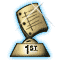Home >> A Tsl >> Archives >> 01 1 >> Area and Perimeter

## Search form

 Home > Teacher Lesson Plans > Archives > Lesson Plan L E S S O N     P L A N The submitter of our highlighted lesson received a \$50 honorarium. See our guidelines to submit your lesson plan!Area and Perimeter Subject: Mathematics, Measurement Grade: 3-5, 6-8 Brief Description This activity helps students discover on their own the formula for learning the area and perimeter of a rectangle and a square. Objectives To enhance student knowledge of measurement. To enhance student thinking and reasoning power. To develop student interest in learning the subject. To help students understand and apply the formulae for determining the area and perimeter of a rectangle and a square. Keywords rectangle, square, area, perimeter, geometry Materials Needed Cut-out of a rectangle divided into number of equal, square regions Cut-out of a square Graph paper Lesson Plan Students learn how to determine the area of a rectangle with the help of graph paper; they count the number of squares on a sample rectangle. Ask students to draw three rectangles on graph paper and to determine their areas by finding the number of squares enclosed. Also ask them to find the rectangles' length and breadth [width] and to create a table that includes that information. The students are to observe the relationships in the information recorded. They observe that the area of a rectangle is equal to the product of its length and breadth [width]. The students know that a square is a rectangle with a pair of adjacent equal sides. They can derive the formula for area of a square by using this fact. Tell the students that the perimeter of a figure is the sum of the measure of all its sides. Then they can derive the formula for perimeter of a rectangle and a square. The students solve the following problem to ensure that they understand the concept and can apply the formula. [Adapt these problems to the U.S. system of measurement.] Find the cost of fencing a rectangular field 32 meters long and 18 meters wide at \$2.25 per meter. Find the cost of cultivating the same field at \$12 per square meter. Assessment Ask students to solve the following problems. The area of a rectangle is 540 centimeters. If its length is 36 centimeters, what is its breadth [width]? Find the area of your study table or desk. What will happen to the area of a square if the length of its side is doubled? Submitted By Narinder Jeet Makkar, Salwan Public School, New Delhi, IndiaSUBMIT YOUR LESSON PLAN 9/14/2001 To help us keep our Lesson Plan Database as current as possible, please e-mail us to report any links that are not working.